# BITSAT Mathematics Vector Algebra MCQs

BITSAT Mathematics Vector Algebra MCQs with answers available in Pdf for free download. The MCQ Questions for BITSAT Mathematics with answers have been prepared as per the latest BITSAT Mathematics syllabus, books and examination pattern. Multiple Choice Questions form important part of competitive exams and BITSAT exam and if practiced properly can help you to get higher rank. Refer to more topic wise BITSAT Mathematics Questions and also download more latest study material for all subjects and do free BITSAT Mathematics Mock Test

## MCQ for BITSAT Mathematics Vector Algebra

BITSAT Mathematics students should refer to the following multiple-choice questions with answers for Vector Algebra in BITSAT. These MCQ questions with answers for BITSAT Mathematics will come in exams and help you to score good marks

### Vector Algebra MCQ Questions with Answers

#### Question: If the position vectors of the vertices A, B, C of a triangle ABC are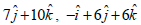andrespectively, the triangle is

• a) Equilateral
• b) Isosceles
• c) Scalene
• d) Right angled and isosceles also

Answer: Right angled and isosceles also

#### Question: For unit vectors b and c and any non-zero vector a, the value of {(a + b) × (a + c)} × (b + c)}. (b + c) is

• a) | a |2
• b) 2 | a |2
• c) 3 | a |2
• d) None of these

• a) 5/7
• b) 6/7
• c) 4/7
• d) 1/2

• a) 15°
• b) 45°
• c) 35°
• d) 60°

#### Question: If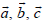are non-coplanar vectors and λ is a real number, then the vectorsare non coplanar for

• a) No value of λ
• b) All except one value of λ
• c) All except two values of λ
• d) All values of λ

Answer: All except two values of λ

#### Question: A vector of magnitude 5 and perpendicular tois

• a)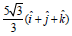• b)• c)• d)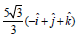• a) i
• b) j
• c) k
• d) 0

#### Question: What is the vector joining the points (3, 1, 14) and (–2, –1, –6) ?

• a)• b)• c)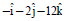• d)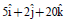#### Question: If= 676 andthenis equal to

• a) 13
• b) 26
• c) 39
• d) None of these

#### Question: Which one of the following is the unit vector perpendicular to both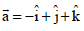and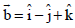?

• a)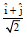• b)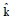• c)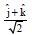• d)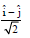#### Question: Letbe non-coplanar unit vectors equally inclined to one another at an acute angle q. Thenin terms of θis equal to

• a)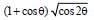• b)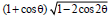• c)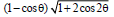• d) None of these#### Question: The dot product of a vector with the vectorsare 0, 5 and 8 respectively. The vector is

• a)• b)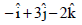• c)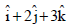• d)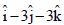• a) 1
• b) -1
• c) 2
• d) -4

#### Question: If the middle points of sides BC, CA & AB of triangle ABC are respectively D, E, F then position vector of centre of triangle DEF, when position vector of A, B, C are respectively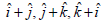is

• a)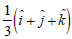• b)• c)• d)#### Question: The angle between any two diagonal of a cube is

• a) 45°
• b) 60°
• c) 30°
• d) tan-1(2 √2)

#### Question: Ifare three unit vectors such that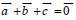whereis null vector, then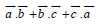is

• a) -3
• b) -2
• c)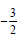• d) 0#### Question: Ifare three non-coplanar vectors, then the value of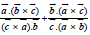is

• a) 0
• b) 2
• c) 1
• d) None of these

• a) 2
• b) -2
• c) -1
• d) -4

#### Question: The unit vector perpendicular to the vectors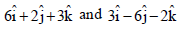is

• a)• b)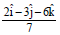• c)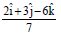• d)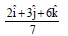#### Question: If a.b = a.c and a × b = a × c, then correct statement is

• a) a || (b – c)
• b)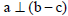• c) a = 0 or b = c
• d) None of these

Answer: a = 0 or b = c

• a) 60°
• b) 90°
• c) 180°
• d) 0°

#### Question: If= 676 andthenis equal to

• a) 13
• b) 26
• c) 39
• d) None of these

#### Question: Which one of the following is the unit vector perpendicular to bothand?

• a)• b)• c)• d)#### Question: With respect to a rectangular cartesian coordinate system, three vectors are expressed as :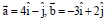andwhere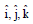are unit vectors, along the X, Y and Zaxis respectively. The unit vectoralong the direction of sum of these vector is –

• a)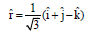• b)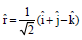• c)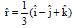• d)#### Question: If the middle points of sides BC, CA & AB of triangle ABC are respectively D, E, F then position vector of centre of triangle DEF, when position vector of A, B, C are respectively i + j, j + k, k + i is –

• a) (1/3) (i + j + k)
• b) (i + j + k)
• c) 2 (i + j + k)
• d) (2/3) (i + j + k)

Answer: (2/3) (i + j + k)